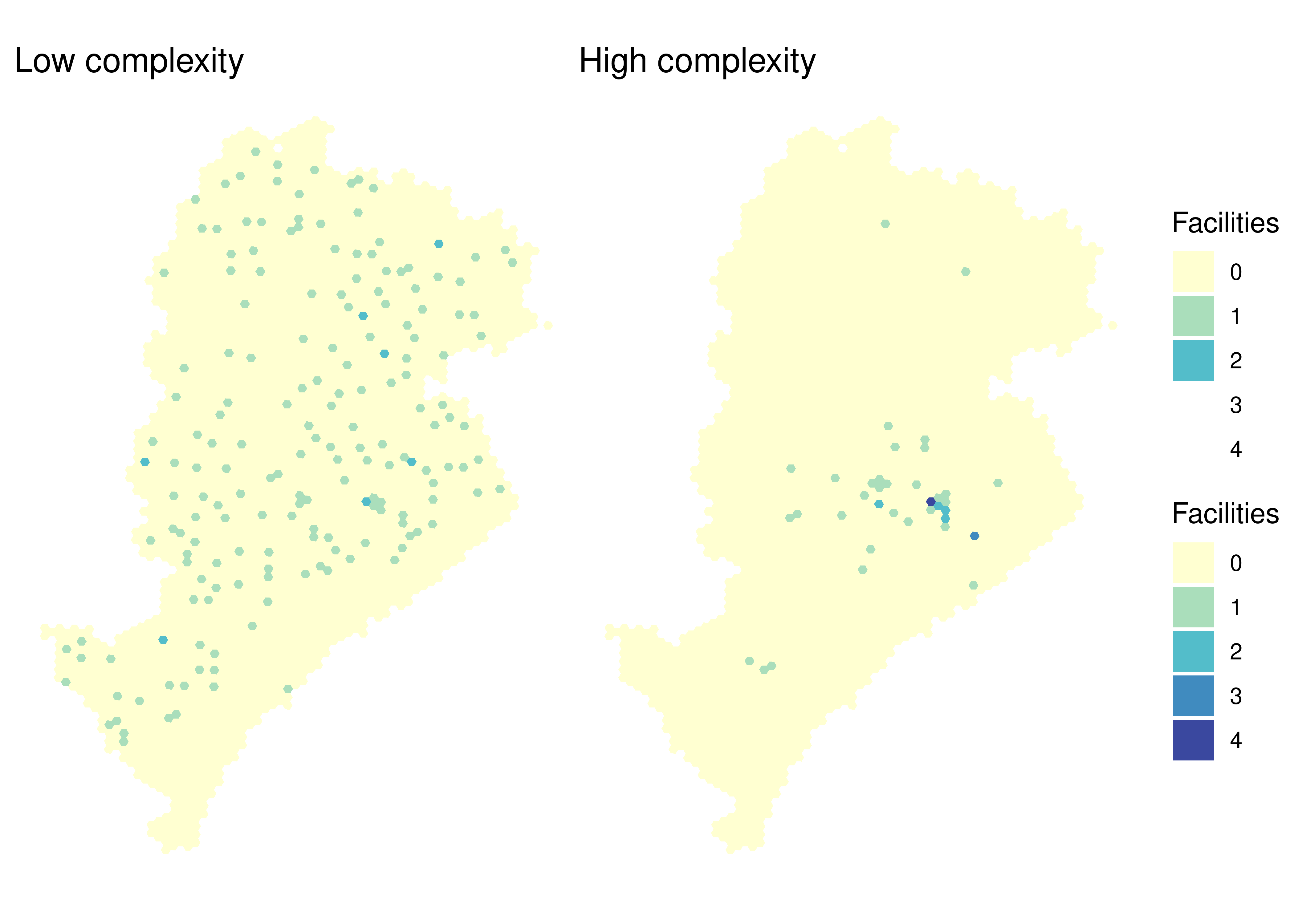# 8Data on the spatial distribution of opportunities

The `{aopdata}` package allows one to download data from 2017, 2018 and 2019 on the spatial distribution of jobs (low, middle and high education), public health facilities (low, medium and high complexity), public schools (early childhood, primary and secondary school levels) and social assistance reference centers (CRAS). This data is available for all cities included in the project.

These datasets can be downloaded with the `read_landuse()` function, which works similarly to `read_population()`. To use it, indicate the city whose data should be downloaded using the `city` parameter, along with the reference year (`year`) and whether to include the spatial information of grid cells or not (`geometry`).

In the example below, we show how to download land use data from 2019 for the city of Belo Horizonte. Please note that this function outputs a table that also includes sociodemographic data.

``````data_bh <- aopdata::read_landuse(
city = "Belo Horizonte",
year = 2019,
geometry = TRUE,
showProgress = FALSE
)

names(data_bh)``````
``````  "id_hex"      "abbrev_muni" "name_muni"   "code_muni"   "P001"
 "P002"        "P003"        "P004"        "P005"        "P006"
 "P007"        "P010"        "P011"        "P012"        "P013"
 "P014"        "P015"        "P016"        "R001"        "R002"
 "R003"        "year"        "T001"        "T002"        "T003"
 "T004"        "E001"        "E002"        "E003"        "E004"
 "M001"        "M002"        "M003"        "M004"        "S001"
 "S002"        "S003"        "S004"        "C001"        "geometry"   ``````

Table 8.1 presents the data dictionary with the description of the table columns (excluding those previously included in the sociodemographic dataset). This description can also be found in the documentation of the function, running the command `?read_landuse` in an R session.

Table 8.1: Description of the columns in the land use dataset
Column Description
year Reference year
id_hex Unique hexagon identifier
abbrev_muni 3-letter abbreviation of municipality name
name_muni Municipality name
code_muni 7-digit municipality IBGE code
T001 Total number of jobs
T002 Number of low-education jobs
T003 Number of middle-education jobs
T004 Number of high-education jobs
E001 Total number of public schools
E002 Number of public early childhood schools
E003 Number of public primary schools
E004 Number of public secondary schools
M001 Total number of students enrolled in public schools
M002 Number of students enrolled in public early childhood schools
M003 Number of students enrolled in public primary schools
M004 Number of students enrolled in public secondary schools
S001 Total number of public health facilities
S002 Number of low complexity public health facilities
S003 Number of mid complexity public health facilities
S004 Number of high complexity public health facilities
C001 Total number of CRAS
geometry Spatial geometry

The following sections show a few examples illustrating how to create spatial visualizations out of this dataset.

## 8.1 Spatial distribution of jobs

In the code below, we load a couple data visualization libraries and configure the map. Columns starting with the letter `T` describe the spatial distribution of jobs in each city. The example shows the spatial distribution of the total number of jobs in each grid cell (variable `T001`) in Belo Horizonte:

``````library(patchwork)
library(ggplot2)

ggplot(data_bh) +
geom_sf(aes(fill = T001), color = NA, alpha = 0.9) +
scale_fill_distiller(palette = "YlGnBu", direction = 1) +
labs(fill = "Jobs") +
theme_void()``````

## 8.2 Spatial distribution of public schools

The columns with information on the number of public schools in each cell begin with the letter `E`. In the example below, we present the spatial distribution of all public schools in Belo Horizonte (variable `E001`).

``````ggplot(data_bh) +
geom_sf(aes(fill = as.factor(E001)), color = NA, alpha = 0.9) +
scale_fill_brewer(palette = "YlGnBu", direction = 1) +
labs(fill = "Schools") +
theme_void()``````

## 8.3 Spatial distribution of public health facilities

The columns with information on the number of public health facilities in each cell begin with the letter `S`. The visualization below compares the spatial distribution of low complexity (`S002`) and high complexity (`S004`) public health facilities.

``````low_complexity <- ggplot(data_bh) +
geom_sf(aes(fill = as.factor(S002)), color = NA, alpha = 0.9) +
scale_fill_brewer(palette = "YlGnBu", direction = 1, limits = factor(0:4)) +
labs(title = "Low complexity", fill = "Facilities") +
theme_void()

high_complexity <- ggplot(data_bh) +
geom_sf(aes(fill = as.factor(S004)), color = NA, alpha = 0.9) +
scale_fill_brewer(palette = "YlGnBu", direction = 1, limits = factor(0:4)) +
labs(title = "High complexity", fill = "Facilities") +
theme_void()

low_complexity + high_complexity + plot_layout(guides = "collect")``````Figure 8.3: Spatial distribution of low complexity and high complexity public health facilities in Belo Horizonte

## 8.4 Spatial distribution of CRAS

Finally, the column `C001` has information on the number of CRAS in each grid cell. The map below shows the spatial distribution of these services in Belo Horizonte.

``````ggplot(data_bh) +
geom_sf(aes(fill = as.factor(C001)), color = NA, alpha = 0.9) +
scale_fill_brewer(palette = "YlGnBu", direction = 1) +
labs(fill = "CRAS") +
theme_void()``````